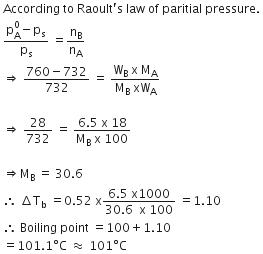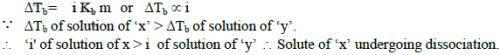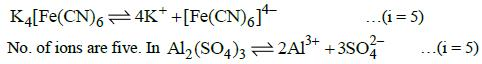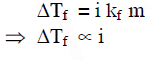# Previous Year Questions (2014-19) - Solutions Notes | EduRev

## NEET : Previous Year Questions (2014-19) - Solutions Notes | EduRev

The document Previous Year Questions (2014-19) - Solutions Notes | EduRev is a part of the NEET Course Chemistry 28 Years Past year papers for NEET/AIPMT Class 12.
All you need of NEET at this link: NEET

Q.1. For an ideal solution, the correct option is :    (2019)
A: Δmix S = 0 at constant T and P
B: Δmix V ≠ 0 at constant T and P
C: Δmix H = 0 at constant T and P
D: Δmix G = 0 at constant T and P
Ans:
C
For ideal solution,
Δmix H = 0
Δmix S > 0
Δmix G < 0
Δmix V = 0

Q.2. The mixture that forms maximum boiling azeotrope is:    (2019)
A: Water + Nitric acid
B: Ethanol + Water
C: Acetone + Carbon disulphide
D: Heptane + Octane
Ans:
A
Solutions showing negative deviation from Raoult's law form maximum boiling azeotrope. Water and Nitric acid → forms maximum boiling azeotrope.

Q.3. If molality of the dilute solutions is doubled, the value of molal depression constant (Kf) will be :-    (2017)
A: halved
B: tripled
C: unchanged
D: doubled
Ans:
C
Kdoes not depend on concentration of solution. It only depends on nature of solvent so it will be unchanged.

Q.4. Which of the following is dependent on temperature?    (2017)
A: Molarity
B: Mole fraction
C: Weight percentage
D: Molality
Ans:
A
Temperature dependent unit is molarity.

Q.5. At 100°C the vapour pressure of a solution of 6.5 g of a solute in 100 g water is 732 mm. If Kb = 0.52, the boiling point of this solution will be :    (2016)
A: 103°C
B: 101°C

C: 100°C
D: 102°C
Ans:
BQ.6. Which of the following statements about the composition of the vapour over an ideal 1 : 1 molar mixture of benzene and toluene is correct ?
Assume that the temperature is constant at 25°C. (Given Vapour Pressure Data at 25ºC, Benzene = 12.8kPa, toluene = 3.85kPa)    (2016)
A: Not enough information is given to make a prediction.
B: The vapour will contain a higher percentage of benzene.
C: The vapour will contain a higher percentage of toluene.The vapour will contain a higher percentage of toluene.
D: The vapour will contain equal amounts of benzene and toluene.
Ans:
B
Due to high partial vapour pressure of Benzene as compare to that of toluene so the mole fraction of Benzene will be higher than that of toluene. As a result the vapour will contain a higher percentage of Benzene.

Q.7. Which one is not equal to zero for an ideal solution ?    (2015)
A: ΔP = Pobserved - PRaoult
B: Δ Hmix
C: ΔSmix
D: Δ Vmix
Ans:
C
For ideal solution, ΔSmix = 0.

Q.8. The boiling point of 0.2 mol kg-1 solution of X in water is greater than equimolal solution of Y in water. Which one of the following statements is true in this case ?    (2015)
A: Y is undergoing dissociation in water while X under goes no change
B: X is undergoing dissociation in water
C: Molecular mass of X is greater than the molecular mass of Y.
D: Molecular mass of X is less than the molecular mass of Y.
Ans:
B
Molality of solution of x = molality of solution of y = 0.2 mol/kg
By elevation in boiling point relationQ.9. Which one of the following electrolytes has the same value of van‘t Hoff‘s factor (i) as that of Al2(SO4)3 (if all are 100% ionised)    (2015)
A: K4[Fe(CN)6]
B: K2SO4
C: K3[Fe(CN)6]
D: Al(NO3)3
Ans:
AQ.10. Of the following 0.10 m aqueous solutions, which one will exhibit the largest freezing point depression?    (2014)
A: Al2 (SO4 )3

B: K2SO4
C: KCl
D: C6H12O6
Ans:
AOffer running on EduRev: Apply code STAYHOME200 to get INR 200 off on our premium plan EduRev Infinity!

16 docs|27 tests

,

,

,

,

,

,

,

,

,

,

,

,

,

,

,

,

,

,

,

,

,

;